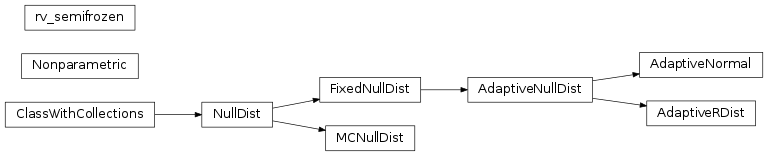# mvpa2.clfs.stats¶

Estimator for classifier error distributions.Functions

 `auto_null_dist`(dist) Cheater for human beings – wraps `dist` if needed with some `match_distribution`(data[, nsamples, loc, ...]) Determine best matching distribution. `nanmean`(x[, axis]) Compute the mean over the given axis ignoring NaNs. `plot_distribution_matches`(data, matches[, ...]) Plot best matching distributions

Classes

 `AdaptiveNormal`(dist, **kwargs) Adaptive Normal Distribution: params are (0, sqrt(1/nfeatures)) `AdaptiveNullDist`(dist, **kwargs) Adaptive distribution which adjusts parameters according to the data `AdaptiveRDist`(dist, **kwargs) Adaptive rdist: params are (nfeatures-1, 0, 1) `FixedNullDist`(dist, **kwargs) Proxy/Adaptor class for SciPy distributions. `MCNullDist`(permutator[, dist_class, measure]) Null-hypothesis distribution is estimated from randomly permuted data labels. `Nonparametric`(dist_samples[, correction]) Non-parametric 1d distribution – derives cdf based on stored values. `NullDist`([tail]) Base class for null-hypothesis testing. `rv_semifrozen`(dist[, loc, scale, args]) Helper proxy-class to fit distribution when some parameters are known